# Influence on the Navigation Channel in Chongqing Reach of the Three Gorges Reservoir Caused by Reservoirs Built Upstream

Influence on the Navigation Channel in Chongqing Reach of the Three Gorges Reservoir Caused by Reservoirs Built Upstream

Jian Chen* Ying Zheng Jun Yan Yan Li

North China University of Water Resources and Electric Power, Zhengzhou Henan 450045, China;

Key Laboratory of Yellow River Sediment Research of Ministry of Water Resources, Zhengzhou Henan 450003, China;

Changjiang Waterway Planning Design and Research Institute, Wuhan Hubei 430011, China.

Corresponding Author Email:
henjian@ncwu.edu.cn
Page:
9-16
|
DOI:
https://doi.org/10.18280/ijht.330202
N/A
|
Accepted:
N/A
|
Published:
30 June 2015
| Citation

OPEN ACCESS

Abstract:

Based on analyzing water and sediment condition and deposition characteristics in Chongqing reach of the Three Gorges Reservoir(TGR), the influence on the deposition and the navigation in Chongqing reach caused by the reservoirs built in the upper stream of the Yangtze River is researched, by using two-dimensional flow and sediment mathematical model. The results indicates that along with river regime adjustment in the early operation stage of TGR and extends upward the backwater end of level 155m, Chongqing reach is the main impeding navigation area in the fluctuating backwater area; Under the combined effects of upstream reservoirs in the stem stream, Chongqing reach will be obviously scoured, making the quantities and thickness of deposition significantly reduced at the original deposition location and even appearing scour, the navigation condition at the stem stream of Chongqing reach has improved a lot. Jinshaqi reach at the junction of the Jia-Ling River is less affected by the construction of upstream reservoir, which making the impeding navigation condition same as that in no upstream reservoir.

Keywords:

Upstream reservoirs, Chongqing reach, erosion and deposition, navigation channel.

1. Introduction

The Three Gorges Reservoir, a large hydro-junction, plays an integrated role in flood control, electricity generation and navigation, the feasibility of which has been done a lot of demonstration. One key problem that directly affects the feasibility is deposition in fluctuating backwater area and the impacts on navigation.

Chongqing reach is located where the Yangtze River and Jia-ling River meet. it is one of the most important shoal reach in fluctuating backwater area of TGR. Based on in-depth analysis of the water and sediment characters in the fluctuating backwater area, some research on main mpeding navigation has been made by many organizations with physical models and some valuable results has been gotten[1-8].

The deposition and navigation in TGR is a complex process affected by upstream incoming water and sediment. With reservoirs design and construction such as Xiluodu reservoir and Xiangjiaba reservoir in the upper stream of the Yangtze River, upstream sediment is intercepted and the incoming sediment of TGR will be further reduced, this will inevitably produce significant impacts on the condition of deposition and waterways of TGR. Analysis of the effect on sediment storage of Chin-sha River and tributary Jia-Ling River on the upstream of Yangtze River was made by Lu Changshi and Di Zhihao, it shown that sediment suspension in fluctuating backwater area can be decreased by 50% by the construction of reservoir on the upstream, in such situation, the suspension sediment deposition in fluctuating backwater area of TGR will be radically improved. Calculations of deposition reduced degree at every shallow shoal in the fluctuating backwater area of TGR is made by Huang Yue with one-dimensional flow and sediment mathematical mode , suggest that Xiluodu Reservoir construction will significantly improve the navigation channel condition in this area.

In this paper, based on two-dimensional flow and sediment mathematical model, the influence of upstream reservoirs on Chongqing reach channel is researched under the condition of reservoirs built in the upper stream of the Yangtze River.

2. Model Foundation

2.1 Fundamental control equation

Flow continuity equation

$\frac{\partial H}{\partial t}+\frac{1}{C_{\xi} C_{\eta}} \frac{\partial}{\partial \xi}\left(h u C_{\eta}\right)+\frac{1}{C_{\xi} C_{\eta}} \frac{\partial}{\partial \eta}\left(h v C_{\xi}\right)=0$     (1)

$\xi$ direction momentum equation

$\frac{\partial u}{\partial t}+\frac{1}{C_{\xi} C_{\eta}}\left[\frac{\partial}{\partial \xi}\left(C_{\eta} u^{2}\right)+\frac{\partial}{\partial \eta}\left(C_{\xi} v u\right)+v u \frac{\partial C_{\eta}}{\partial \eta}-v^{2} \frac{\partial C_{\eta}}{\partial \xi}\right]= -g \frac{1}{C_{\xi}} \frac{\partial H}{\partial \xi}+f v-\frac{u \sqrt{u^{2}+v^{2}} n^{2} g}{h^{4 / 3}}+ \frac{1}{C_{\xi} C_{\eta}}\left[\frac{\partial}{\partial \xi}\left(C_{\eta} \sigma_{\xi \xi}\right)+\frac{\partial}{\partial \eta}\left(C_{\xi} \sigma_{\eta \xi}\right)+\sigma_{\xi \eta} \frac{\partial C_{\xi}}{\partial \eta}-\sigma_{\eta \eta} \frac{\partial C_{\eta}}{\partial \xi}\right]$    (2)

$\eta$ direction momentum equation

$\frac{\partial_{v}}{\partial_{t}}+\frac{1}{C_{\xi} C_{\eta}}\left[\frac{\partial}{\partial \xi}\left(C_{\eta} v u\right)+\frac{\partial}{\partial \eta}\left(C_{\xi} v^{2}\right)+u v \frac{\partial C_{\eta}}{\partial \xi}-u^{2} \frac{\partial C_{\xi}}{\partial \eta}\right]= -g \frac{1}{C_{\eta}} \frac{\partial H}{\partial \eta}-f u-\frac{v \sqrt{u^{2}+v^{2}} n^{2} g}{h^{4 / 3}}+ \frac{1}{C_{\xi} C_{\eta}}\left[\frac{\partial}{\partial \xi}\left(C_{\eta} \sigma_{\xi \eta}\right)+\frac{\partial}{\partial \eta}\left(C_{\xi} \sigma_{\eta \eta}\right)+\sigma_{\eta \xi} \frac{\partial C_{\eta}}{\partial \xi}-\sigma_{\xi \xi} \frac{\partial x_{\xi}}{\partial \eta}\right]$   (3)

Where $\xi$ ,$\eta$ are two coordinate of curvilinear orthogonal coordinate system. Where $u$ ,$v$ is $\xi$,$\eta$ direction velocity. Where $h$ is water depth. Where $H$ is water level. Where $f$ is coriolis coefficient. Where $C_{\xi}$ ,$C_{\eta}$ are lame coefficient in curvilinear orthogonal coordinate system.

$C_{\xi}=\sqrt{x_{\xi}^{2}+y_{\xi}^{2}}$

$C_{\eta}=\sqrt{x_{\eta}^{2}+y_{\eta}^{2}}$

Where$\sigma_{\xi \xi}, \sigma_{\xi \eta}, \sigma_{\eta \xi}, \sigma_{\eta \eta}$ are turbulent stress.

$\sigma_{\xi \xi}=2 v_{t}\left[\frac{1}{C_{\xi}} \frac{\partial u}{\partial \xi}+\frac{v}{C_{\xi} C_{\eta}} \frac{\partial C_{\xi}}{\partial \eta}\right]$

$\sigma_{\eta \eta}=2 v_{t}\left[\frac{1}{C_{\eta}} \frac{\partial}{\partial \eta}+\frac{u}{C_{\xi} C_{\eta}} \frac{\partial C_{\eta}}{\partial \xi}\right]$

$\sigma_{\xi \eta}=\sigma_{\eta \xi}=v_{t}\left[\frac{C_{\eta}}{C_{\xi}} \frac{\partial}{\partial \xi}\left(\frac{v}{C_{\eta}}\right)+\frac{C_{\xi}}{C_{\eta}} \frac{\partial}{\partial \eta}\left(\frac{u}{C_{\xi}}\right)\right]$

Where$V_{t}$ is turbulent.

Non-equilibrium sediment transport equation of suspended load

Considering non-uniform flow sediment concentration, the equation is

$\frac{\partial h S_{L}}{\partial t}+\frac{1}{C_{\xi} C_{\eta}}\left[\frac{\partial}{\partial \xi}\left(C_{\eta} h u S_{L}\right)+\frac{\partial}{\partial \eta}\left(C_{\xi} h v S_{L}\right)\right]=$

$\frac{1}{C_{\xi} C_{\eta}}\left[\frac{\partial}{\partial \xi}\left(\frac{\varepsilon_{\xi}}{\sigma_{s}} \frac{C_{\eta}}{C_{\xi}} \frac{\partial h S_{L}}{\partial \xi}\right)+\frac{\partial}{\partial \eta}\left(\frac{\varepsilon_{\eta}}{\sigma_{s}} \frac{C_{\xi}}{C_{\eta}} \frac{\partial h S_{L}}{\partial \eta}\right)\right]+\alpha_{L} \omega_{L}\left(S_{L}^{*}-S_{L}\right)$    (4)

Where S_{L}^{*} is someone group sediment carrying capacity. Where P_{S L}^{*} is sediment carrying capacity of someone group. Where \omega_{L} is settling velocity of someone group. Where \alpha_{L} is saturation recovery of someone group.

Non-equilibrium sediment equation of bed load

$\frac{\partial h S_{b L}}{\partial t}+\frac{1}{C_{\xi} C_{\eta}}\left[\frac{\partial}{\partial \xi}\left(C_{\eta} h u S_{b L}\right)+\frac{\partial}{\partial \eta}\left(C_{\xi} h v S_{b L}\right)\right]= \frac{1}{C_{\xi} C_{\eta}}\left[\frac{\partial}{\partial \xi}\left(\frac{\varepsilon_{\xi}}{\sigma_{b}} \frac{C_{\eta}}{C_{\xi}} \frac{\partial h_{b L}}{\partial \xi}\right)+\frac{\partial}{\partial \eta}\left(\frac{\varepsilon_{\eta}}{\sigma_{b}} \frac{C_{\xi}}{C_{\eta}} \frac{\partial h S_{b L}}{\partial \eta}\right)\right]+\alpha_{b L} \omega_{b L}\left(S_{b L}^{*}-S_{b L}\right)$    (5)

Where $S_{b L}^{*}$ is the total depth sediment concentration converted from sediment carrying capacity, $S_{b L}^{*}=g_{b L}^{*} /(\sqrt{u^{2}+v^{2}} h)$. Where $g_{b L}^{*}$ is unit width sediment transport rate. Where $S_{b L}$ is full depth sediment concentration converted from someone group sediment carrying capacity, $S_{b L}=g_{b L} /(\sqrt{u^{2}+v^{2}} h)$ . Where $\alpha_{b L}$ is someone group sediment saturation recovery coefficient. Where $\omega_{b L}$ is someone group sediment settling velocity.$\sigma_{b}=1$.

Formula of sediment carrying capacity and gradation of sediment carrying capacity

Sediment carrying capacity is related with $u, h, \omega$ . A curve showing the relationship between $S$ and $\bar{u}^{3} / g h \omega$ is drown based on the observation data under the vertical flurial equilibrium. The curve gives a formula of two-dimensional sediment carrying capacity:

$S_{*}=K\left(\frac{\bar{u}^{3}}{g h \omega}\right)^{m}$   (6)

Where $K, m$ adjusted on the observation data of water and sediment.

Li Yitian method is adopted. Considering the effects of flow conditions and sediment combination, the relationship between sediment carrying capacity gradation $P_{* k}$ and sediment gradation $P_{b k}$ is found through analysis:

$P_{* k}=P_{b k} \frac{\frac{1-A_{k}}{\omega_{k}}\left(1-e^{-\frac{6 \omega_{k}}{k u_{*}}}\right)}{\sum_{k=1}^{n} P_{b k} \frac{1-A_{k}}{\omega_{k}}\left(1-e^{-\frac{6 \omega_{k}}{k u_{*}}}\right)}$

$A_{k}=\frac{\omega_{k}}{\frac{\sigma_{v}}{\sqrt{2 \pi}} e^{-\frac{\omega_{k}^{2}}{2 \sigma_{V}^{2}}}+\omega_{k} \Phi\left(\frac{\omega_{k}}{\sigma_{v}}\right)}$  (7)

Where $u_{*}$ is friction velocity. Where $\kappa$ is Karman constant. Where $n$ is the number of groups. Where $\sigma_{v}$ is vertical turbulence intensity. $\sigma_{v}=u_{*}$ . $\Phi\left(\frac{\omega_{k}}{\sigma_{v}}\right)$ can be solved with numerical integration.

Bed load transport rate is related with $u, h, d, \omega$. There are many formula about bed load transport rate. Calculation results can be very different between different formula. Modified Dou Guoren formula is adopted here :

$g_{b L}=\frac{k_{1}}{C_{0}^{2}} \frac{\rho_{s} \rho}{\rho_{s}-\rho}\left(u-u_{c}\right) \frac{\bar{u}^{3}}{g \omega}$    (8)

Where $U_{c}$ is incipient velocity corresponding the grain size of sediment. $C_{0}=\frac{h^{1 / 6}}{n \sqrt{g}}$ . Where $k_{1}$ is empirical coefficient. The gradation bed load transport rate is the result of total bed load transport rate multiply by bed load gradation.

2.2 Model Solution

Numerical solution

Equation(1)～(5)are similar. So all they can be converted to this general style:

$C_{\xi} C_{\eta} \frac{\partial \psi}{\partial}+\frac{\partial\left(C_{\eta} u \psi\right)}{\partial \xi}+\frac{\partial\left(C_{\xi} v \psi\right)}{\partial \eta}=\frac{\partial}{\partial \xi}\left(\Gamma \frac{C_{\eta}}{C_{\xi}} \frac{\partial \psi}{\partial \xi}\right)+\frac{\partial}{\partial \eta}\left(\Gamma \frac{C_{\xi}}{C_{\eta}} \frac{\partial \psi}{\partial \eta}\right)+C$   (9)

A general program written to(9)can solve all control equations. Where $\Gamma$ is diffusion coefficient. Where $C$ is source term.

Staggered grid is adopted, making $u, v, h$ distribute at a same grid, inlet boundary and outlet boundary across vertical velocity computational point, fixed boundary across transverse velocity computational point. Density of grid is depended on physical quantity variation degree. Control volume method is adopted to discrete equation. Computation area is partitioned to series of consecutive volume without superposition. Every control volume contain a computation node. The unknown number of discrete equation is dependent variable $\psi$ .Control section is dispute at the middle of adjacent node on the assumption that physical quantity change in power function law. SIMPLEC is adopted here.

Definite conditions

Initial water level field is available from linear interpolating on distance between cross sections. When computational area is long, linear interpolation in subsection using water surface profile method.

To flow velocity field, $\eta$ direction $v=0$ , $\xi$ direction $u$ be calculated from maning formula. Bank boundary water level is given by normal condition($\frac{\partial}{\partial n}=0$). Bank boundary velocity is 0, entrance velocity and exit water level are given by ($\frac{\partial}{\partial n}=0$)

3. Model Verification

3.1 Verification conditions

Verification is based on 1:5000 real topography from Hydrographic and Water Resources Survey Bureau in Dec, 2002. These hydrologic sections location are shown in Figure.1. Water level profile verification and velocity verification is done at Cuntan station, Lijiatuo station, and Liziba station. Movable bed verification is done at Jiulongpo reach, Chaotianmen reach, and Jinshaqi reach.

Water and sediment data of Dadukou station and Shimahe station in 2003 is adopted as inlet boundary data. Outlet boundary water level is provided by one-dimensional computation.

## 1.png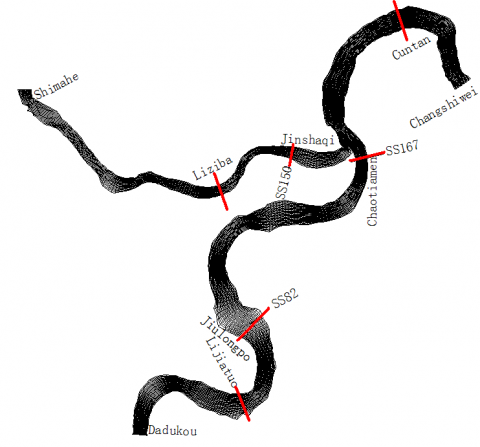Figure 1. Layout of cross section and grids of calculated region

3.2 Analysis of verification results

Three different discharge data in 1986 and flood happened in Jul.16, 1981 are adopted to verify water level profile. The error of all the seven computational water level is about5cm.

Velocity verification is based on the observation data in Sep.Dec.1986, Cuntan station, Lijiatuo station and Liziba station with two different discharge. The results is shown in Table.1. The relative error of section mean velocity is less than 4% .Vertical mean velocity 9%. These indicate that simulation results agree well with measurements and the model is reliable.

Table 1. Chongqing reach velocity verification results

 Section conditions velocity (QShimahe=856m3/s, QDadukou=5334m3/s) velocity  (QShimahe=1690m3/s, QDadukou=18000m3/s) Cuntan start point distance (m) 73.1 130.1 179.1 240.1 290.1 350.9 414.1 average 105.1 211.1 272.1 351.1 446.1 516.1 641.1 average prototype 1.77 2.34 2.57 2.42 2.22 1.75 1.43 2.07 1.99 2.87 2.79 2.64 2.45 2.28 1.87 2.41 model 1.70 2.50 2.61 2.54 2.15 1.71 1.31 2.07 2.13 2.96 2.85 2.61 2.39 2.30 1.76 2.42 error -3.95 6.84 1.56 4.96 -3.15 -2.29 -8.39 0.14 7.04 3.14 2.15 -1.14 -2.45 0.88 -5.88 0.65 Lijiatuo start point distance (m) 245 305 365 425 485 545 595 average 150 225 300 375 450 510 585 average prototype 1.91 2.25 2.36 2.44 1.86 1.51 1.54 1.98 2.3 2.82 2.86 2.86 2.75 2.71 2.86 2.75 model 1.79 2.21 2.42 2.49 1.82 1.62 1.63 2.00 2.15 2.80 2.91 2.91 2.79 2.75 2.92 2.75 error -6.28 -1.78 2.54 2.05 -2.15 7.28 5.84 0.79 -6.52 -0.71 1.75 1.75 1.45 1.48 2.10 0.37 Liziba start point distance (m) 153 203 261 307 371 average 119 183 293 341 391 452 average prototype 1.59 1.43 0.79 0.72 0.63 1.03 0.5 0.57 0.55 0.51 0.40 0.32 0.48 model 1.65 1.51 0.82 0.73 0.64 1.07 0.47 0.60 0.57 0.49 0.37 0.30 0.47 error 3.77 5.59 3.80 1.39 1.59 3.68 -6.00 5.26 3.64 -3.92 -7.50 -6.25 -1.75
4. Effects on Navigation Channel At Chongqing Reach with Reservoirs Built Upstream

Calculations is made about the influence of the navigation channel of Chongqing reach caused by the reservoirs built in the upper stream of the Yangtze River such as Xiluodu Reservoir and Xiangjiaba Reservoir by using two-dimensional flow and sediment mathematical model. the range of calculations are shown in Figure.1. The inflow of the calculation information is based on measured data of water and sediment from 1961 to 1970 of Dadukou station and Shimahe station. The plan of declining the amount of sediments under the condition of the reservoirs built upstream is shown in reference , and Changshiwei station is the outflow boundary, it’s  water level is provided by the one-dimensional flow model. The plan of water level in front of dam is given bellow: From June 16th, 2003 to September 30th, 2006, it adopt the 139m-135m application; From October 1th, 2006 to September 30th, 2007, it adopt 156m-135m-140m application; From October 1th, 2007 to December 31th, 2013, it accords to 172m-144m-152m application; At the Beginning of 2014, it adopt 175m-145m-155m application.

4.1 Distribution of scour and silting of sediment and change trend

When TGR stores water, because of backwater effects, deposition accumulated appears in the Chongqing reach. Table.2 shows the deposition accumulated of main shoal and whole reach every ten years of TGR, with reservoirs built upstream, the negative deposition amount means erosion.

From the Table.2, with TGR operating, the deposition volume at Chongqing reach increases year by year: 2486×104 m3 silt in the 20th year, 3513×104 m3 in the 50th year, and 5089×104 m3 in the 100th year, increasing apparently. Figure.2 shows deposition distribution at Chongqing reach, From which it can seen that the deposition at Chongqing reach, at the end of the 100th year , is mainly vertically distributed in wider shallow Shoals like Jiulongpo reach, Jinshaqi reach and so on. And the deposition mainly horizontally distributed at beach sides, recirculation zone or other parts.

From 2011, affected by the upstream reservoirs, main stream trends to erosion. From Table.2, at the beginning of the 10th year of the reservoir operation, the deposition volume of Jiulongpo reach, Chaotianmen reach in Chongqing reach decreases rapidly and the reservoir enter the stage of erosion, indicating the strong effects on Chongqing reach by upstream reservoir. Because of the combined operation of two upstream reservoirs, sediment delivery ratio in a long run maintains a low level, and therefore, it was that  until the 70th year, with sediment delivery ratio becoming higher and higher and the impacts of the riverbed armoring that the reach turns to silt up from scouring. But until the end of 100th year, it is still in the stage of erosion, the upstream reservoir ensuring the entire Yangtze River away from deposition in 100 years. Because incoming water and sediment condition is the same as those in without upstream reservoir, at the same time, not taking the operation effects of upstream Tingzikou Reservoir into account, the deposition development of Jinshaqi reach in Jia-Ling River and the amount of deposition have little difference.  Figure.3 shows the distribution of deposition under the condition of the construction of reservoir on the upstream at the end of the 100th year. From the picture, with the operation of reservoir for 100 years, although the river was always in the stage of erosion, some deposit still appears in backwater area and shallow shoals. If the upstream reservoir does not exist, the range of the deposition is obviously compressed. The influence on the development of deposition is not obvious to tributaries under the condition of the construction of upstream reservoir. Jinshaqi reach in Jia-Ling River is obviously silting up. The position of deposition is basically the same and the amount of that slightly decreases without upstream reservoir. However, if considering the effect of tributary reservoir preventing sand, the deposition condition in the Jinshaqi reach is likely to be improved in quite a long time.

Table 2. Deposition amounts in different reaches of Chongqing reach(104m3)

 Year Jinshaqi reach Chaotianmen reach Jiulongpo reach Chongqing reach No reservoir built  upstream Reservoir built upstream No reservoir built  upstream Reservoir built upstream No reservoir built  upstream Reservoir built upstream No reservoir built  upstream Reservoir built upstream 10th 506 506 151 151 293 293 2197 2197 20th 569 577 170 -107.9 329 -567 2486 -3374.5 30th 653 643 193 -123 375 -585 2822 -3680 40th 728 708 215 -128.3 416 -576 3151 -3720 50th 815 774 237 -126.8 463 -562 3513 -3640 60th 911 842 260 -125.3 515 -562 3910 -3550 70th 998 903 285 -123.8 561 -558 4311 -3409 80th 1104 968 310 -120 618 -540 4696 -3300 90th 1170 1022 326 -114.7 656 -526 4981 -3203.5 100th 1182 1075 330 -104.2 663 -510 5089 -3071

## 2.png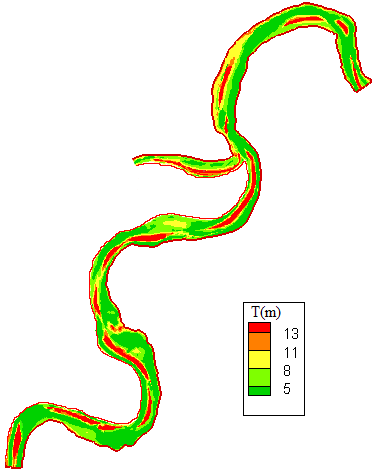Figure2. Deposition thickness distribution in Chongqing reach without reservoir building upstream (m)

## 3.png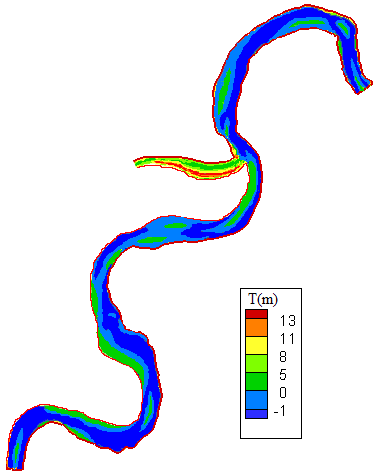Figure 3. Deposition thickness distribution in Chongqing with reservoir building upstream(m)

4.2 Sediment deposition situation and the effect on navigation channel in key shoals

The following analysis focuses on the sediment deposition and possible effect on navigation channel at major shoals in Chongqing reach with TGR storage and the construction of upstream reservoir.

Jiulongpo reach

Without upstream reservoirs, in the first five years after storage, the reach having not been affected by backwater, change laws of scouring and silting is little different from that in natural condition. In 2007, when the scheme (172m and 175m storage) is put into use, due to the delay of sediment transported time and the decrease of sediment transported volume, the reach produced accumulative deposition, the deposit position being the same as that in natural condition. With the run time increasing, marginal bank gradually accumulates, making channel width narrow and depth shallow. Marginal banks are exposed in storage falling stage, especially when departing abundance of sediment year and entering the low-water year, leading more serious impeding navigation conditions. For example, the storage period after flood season in the 28th year (in 1968, the year with abundance of sediment) and falling stage before flood season in the 29th year , there are 15 days in which the water depth is less than 3.5m at apron space and the waters width is less than 200m, leading certain impacts on the normal navigation. Marginal bank gradually increased to 200~300m in the middle storage period, leading certain impact on navigation. In later stage, marginal bank expands to 250~400m, seriously affecting the terminal operation. After abundance of sediment year, the phenomenon of inverted trough will appear, making flow unstable. And the number of impeding navigation days is about 35.

The change law of scour and silting in the condition of the construction of upstream reservoir has obvious changes compared with that in no reservoir. The whole river is in the scour stage. And the deposition trend increases and scouring trend decreases in many original deposit position. As we can see from Table.2, since the 11th year of TGR put into use, deposition accumulated in Jiulongpo reach significantly decreases, showing scouring trend and very strong. In the 20th year, compared with natural condition, the deposition volume of Jiulongpo reach reaches 567×104m3. With incoming sediment from upstream increasing and the riverbed coarsened, the reach gradually silts up in the 80th year. However the volume accumulated of erosion is still 540×104 m3 at the end of the 80th year. Figure.4(a) shows that in the 78th year, bench sections compare of the SS82 section under the condition that weather there is the construction of upstream reservoir in Jiulongpo reach. From the Figure.4(a), original deposition position on the left bank is still in the stage of deposition, however, the thickness reducing significantly in two different cases. In flood season apparent erosion appears at deposition position originally changed slightly or some deposition positions. The main reason for this phenomenon is that flow goes straight in flood season. Under the condition of the construction of upstream reservoir, sediment concentration decreases, compared to the natural condition, sediment-carrying capacity getting surplus. Erosion trend becomes stronger in the main channel during flood season. Sediment deposition still exists in backwater area on the left bank, due to the slow flow. And, due to sediment concentration reduced, the volume and range of deposition significantly decreases in the flood season. At the same time, in the following year, erosion becomes relatively significantly because of incoming sediment reduction form upstream. For example, the 28th year is abundant incoming sediment year, the volume of left bank deposition amounting to 450×104 m3 under the condition of no reservoir on the upstream and 380×104 m3 flushing sediment after the flood season. Moreover, left branch deposition amounted to 180×104 m3 under the condition of reservoir on the upstream, and sediment scoured volume  is up to 165×104 m3 after the flood season.

Therefore, under the condition of building reservoir on upstream, Jiulongpo reach is in the state of erosion overall, especially main channel scoured deeply and making no impeding navigation happen in 100 years in that reach.

Chaotianmen reach

Zhuerqi area and Yueliangqi area are important shoal in Chaotianmen reach. The scheme (175m) implemented in early days under the condition of no reservoir on the upstream, in the influence of backwater, channel conditions in the after flood to next flood season has improved, right deep groove depth more than 4.0m and the minimum width navigation being 120m; With the run time increasing, the influence of backwater has been increasing year by year, leading sediment accumulated increasing and the Zhuerqi area expanding each year. When Jia-Ling River enters mass flow year, the confluence produces jacking phenomenon, deposition increased in the early time and unsmooth scour. latent moraines are exposed  in the water level falling period, having an adverse impact on navigation; In later days, the Zhuerqi area is silting up strongly, even no way for flowing at left branch, connected to Yueliangqi area that is silting also strongly, 150~250m beach width, impacting the wharf operation near the left bank. and in falling period impeding navigation situation will appear. When flow volume is mass in the stem river and the branch jacking phenomenon is not obvious, the beach tends to erosion. The navigation condition is close to natural in flood season, due to storage after this season, navigation conditions are improved in a way.

Under the condition of the construction of upstream reservoir, The Chaotianmen reach overall tends to erosion, original deposition position obviously decreasing. From data in Table.2, from the 11th year, the Chaotianmen reach is in strong erosion the stage, in accordance with that in Jiulongpo reach. In the 40th year of reservoir operation, relative to the natural condition, cumulative erosion volume of the whole Chaotianmen reach amounted to 128.3×104 m3. Then with upstream incoming sediment increasing and bed coarsening, the river is gradually turn to deposition, but until the 80th year, the Chaotianmen reach is still in the erosion stage, the volume amounting to 120×104 m3. From Figure.3 and Figure.4(b), the deposition mainly appears in the left beach face of Yueliangqi, however, deposition thickness significantly reduced under the condition of construction of upstream reservoir, right deep groove continuing scouring deeply. Impeding navigation situation hardly happens in this reach in 100 years under the condition of the construction of upstream reservoir.

## 4a.png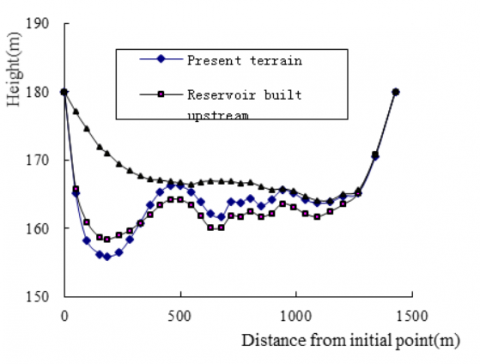## 4b.png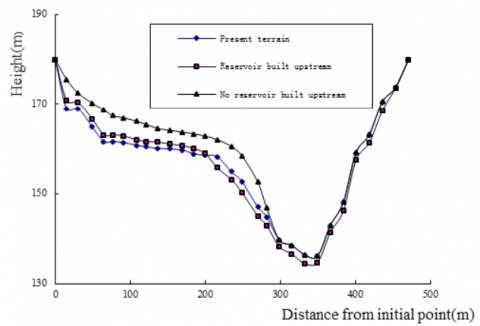## 4c.png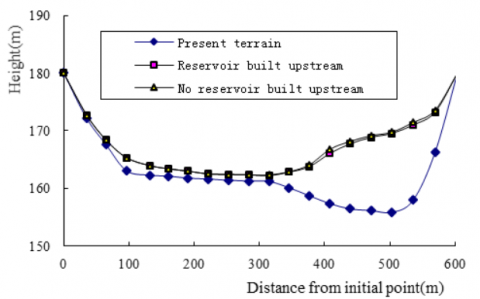Figure.4 Change of typical cross sections with and without reservoir construction upstream

Jinshaqi reach

Under the condition of no upstream reservoir built, the deposition appears in the right main channel of the upstream relaxing reach, right channel’s deposition is higher, the formation of beach in the jetty, gradually downward. Deposition in right channel is characterized silting up within this year and scouring in the next year, however, the erosion not complete, making beach gradually developing. Such phenomenon expresses obviously.

Due to Jinshaqi reach is in the tributary of Jia-Ling River, under the condition of upstream reservoir built in the Yangtze River, incoming water and sediment condition of the Jinshaqi reach was not affected, only the downstream backwater extent is affected. Scour and silting changes in this reach under the condition of upstream reservoir built in the Yangtze River is little different from that without upstream reservoir. From Figure.3 and Figure.4 (c) can be seen, after the upstream reservoir is built in the Yangtze River, the deposition is still mainly occurred in the right main channel of the upstream relaxing reach, right channel aggradation, the formation of beach in the jetty, gradually downward, deposition thickness under the condition of upstream reservoir built at a little smaller than that of no reservoir. In the middle and later 175m storage periods, deposition developing significantly strong in right Chaotianmen apron space, the beaches appears in front of No.1 and No.2 Wharfs,  the width of which is  100~250m; No.3 and No.4  also  have some problems that navigation depth is less than 3.0m from January to February and the navigation width is less than 100m. The development of bank deposit, may cause the mainstream middle, Jinshaqi shoal cutting, so be prepared as soon as possible to control, otherwise it will have a greater impact on navigation.

Generally, the moraine deposition volume under the condition of reservoir built on the upstream decreases slightly, compared with that without reservoir.

5. Conclusion

The results are given below, through the calculation analysis of water and sediment changes, as well as navigation conditions in fluctuating backwater area of Gorges reservoir.

(1) Under the condition of no reservoir built on the upstream, due to raising of water level in the Chongqing reach in the storage period after flood season, original sediment transported laws will be disturbed, leading accumulated deposition in the early reservoir operated period. With the run time of reservoir increasing, part of the beach reach will produce the problem of impeding navigation.

(2) With the construction of reservoirs in the upper stream of the Yangtze River, incoming flow’s sediment carrying capacity is surplus in TGR. With TGR retaining water in 100 years, the whole Chongqing reach in the Yangtze River is eroded, impeding navigation doesn’t appear, but the deposition in Jinshaqi reach of Jia-Ling River has a little difference with that no reservoir built upstream in the upper stream of the Yangtze River.

Acknowledgement

The study was partially supported by Open fund of Key Laboratory of Yellow River Sediment Research of Ministry of Water Resources (2015006) and the Support Plan for Young Talented Person with Scientific and Technological Innovation(70453).

References

1. Nanjing Hydraulic Research Institute, Research and application of 2-D sediment mathematical model of backwater region of the Three Gorges Reservoir, Report of special research on the key technology of Three Gorges Project on the Yangtze River sediment and navigation, Nanjing, China, pp: 1147～1166, 1993.

2. Wuhan Institute of water conservancy and electric power, Research and preliminary application on sediment mathematical model(one-dimensional, one-dimensional and two-dimensional nested) of the backwater region of the Three Gorges reservoir, Report of special research on the key technology of Three Gorges Project on the Yangtze River sediment and navigation, Wuhan, China, pp: 1167～1219, 1993.

3. Department of researching on hydroelectric and sediment of Tsinghua University, Total experimental report on the sediment problems of Chongqing reach of Three Gorges Reservoir, Report of special research on the key technology of Three Gorges Project on the Yangtze River sediment and navigation, Beijing, China, pp: 152～208, 1993.

4. China Institute of Water Resources and Hydropower Research, Summary report of the Three Gorges Reservoir test on the sediment problem of Chongqing Reach, Report of special research on the key technology of Three Gorges Project on the Yangtze River sediment and navigation, Beijing, China, pp: 209～238, 1993.

5. Yangtze River Scientific Research Institute, Comprehensive experimental report on the sediment problems of Chongqing reach of the Three Gorges reservoir, Report of special research on the key technology of Three Gorges Project on the Yangtze River sediment and navigation, Wuhan, China, pp: 239～263, 1993.

6. Yangtze River Scientific Research Institute, Comprehensive study report of sediment model test in Tongluoxia reach of backwater area of the Three Gorges reservoir, Report of special research on the key technology of Three Gorges Project on the Yangtze

River sediment and navigation, Wuhan, China, pp: 264～297 1993,.

7. Tianjin Research Institute for Water Transport Engineering, M.O.T. the general report of sediment model test of Wangjiatan reach in backwater region of Three Gorges reservoir, Report of special research on the key technology of Three Gorges Project on the Yangtze River sediment and navigation, Tianjin, China, pp: 298～335, 1993.

8. Wuhan Institute of Water Conservancy and Electric power, the general report of sediment model test of Qingyanzi reach in backwater region of Three Gorges reservoir, Report of special research on the key technology of Three Gorges Project on the Yangtze River sediment and navigation, Wuhan, China, pp: 1167～1219, 1993.

9. Lu Changshi, Di Zhihao, Measures to improve the deposition in varying backwater zone of the Three Gorges project, Journal of hydroelectric engineering, Beijing, China, pp:89～96, 1998.

10. Huang Yue, Huang Yuling, Research and practice in environment protection for Three Gorges Project, China Three Gorges Construction, Wuhan, China, pp: 16～18, 2002.

11. Li Yitian, 2-D numerical model of River sediment, Wuhan of Water Resources and Electric Power doctoral dissertation, Wuhan, China, pp: 116～117, 1987.

12. Zhang Ruijin, River sediment dynamics, China water power press, Wuhan, China, pp: 66～68, 1989.

13. Wuhan University, Calculation of deposition and influence on navigation of Three Gorges Reservoir, Wuhan, China, pp: 74～76, 2006.